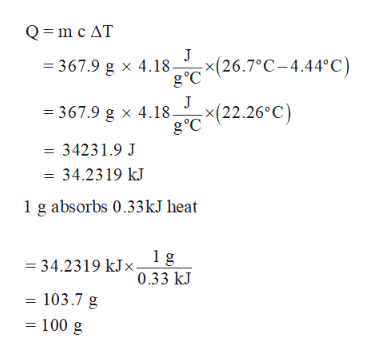# When ice melts, it absorbs 0.33 kJ per gram.How much ice is required to cool a 13.0 oz drink from 80 ∘F to 40 ∘F, if the heat capacity of the drink is 4.18 J/g∘C? (Assume that the heat transfer is 100 % efficient.)        Express your answer using two significant figures.

Question
733 views

When ice melts, it absorbs 0.33 kJ per gram.

How much ice is required to cool a 13.0 oz drink from 80 ∘F to 40 ∘F, if the heat capacity of the drink is 4.18 J/g∘C? (Assume that the heat transfer is 100 % efficient.)
check_circle

Step 1

First, the grams of drink is determined and then the given temperature in Fahrenheit is converted into Celsius as follows,

Step 2

Finally, the amount of ice require...help_outlineImage TranscriptioncloseQ m c AT 367.9 g x 4.18(26.7°C-4.44°C) g°C J x(22.26°C) 367.9 g x 4.18. g°C 34231.9 J - 34.2319 kJ 1 g absorbs 0.33kJ heat 1g = 34.2319 kJ0.33 kJ 103.7 g 100 g fullscreen

### Want to see the full answer?

See Solution

#### Want to see this answer and more?

Solutions are written by subject experts who are available 24/7. Questions are typically answered within 1 hour.*

See Solution
*Response times may vary by subject and question.
Tagged in

### Chemistry